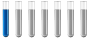## Orca - calculations, Gibbs energy, solvent

Chemistry and homework help forum.

Organic Chemistry, Analytical Chemistry, Biochemistry, Physical Chemistry, Computational Chemistry, Theoretical Chemistry, High School Chemistry, Colledge Chemistry and University Chemistry Forum.

Share your chemistry ideas, discuss chemical problems, ask for help with scientific chemistry questions, inspire others by your chemistry vision!

Please feel free to start a scientific chemistry discussion here!

Discuss chemistry homework problems with experts!

Ask for help with chemical questions and help others with your chemistry knowledge!

Moderators: Xen, expert, ChenBeier

astor
NewbiePosts: 1
Joined: Thu Nov 18, 2021 4:13 pm

### Orca - calculations, Gibbs energy, solvent

Hi,

I would like to ask you a question about basics of computational chemistry.

I need to calculate free Gibbs energy of the reaction in solvent, so I need to calculate data for reactants and products. But the point is that teacher told us not to calculate the Gibbs energy of molecules in solvent, but to calculate single point energies in solvent and add some contributions to this energy. One should be contribution from frequency calculation in vacuum, and what about the second contribution for the solvent? What calculation should I do and what number I need from this calculation? I do calculations in Orca.
Thank you